# 2020 seminars

## 07/04/2020, Tuesday, 16:00–17:00 Europe/Lisbon Room P1, Mathematics Building — OnlineSusanna Terracini, Università di Torino
Pattern Formation Through Spatial Segregation

Reaction-diffusion systems with strong interaction terms appear in many multi-species physical problems as well as in population dynamics. The qualitative properties of the solutions and their limiting profiles in different regimes have been at the center of the community's attention in recent years. A prototypical example is the system of equations $\left\{\begin{array}{l} -\Delta u+a_1u = b_1|u|^{p+q-2}u+cp|u|^{p-2}|v|^qu,\\ -\Delta v+a_2v = b_2|v|^{p+q-2}v+cq|u|^{p}|v|^{q-2}v \end{array} \right.$ in a domain $\Omega\subset \mathbb{R}^N$ which appears, for example, when looking for solitary wave solutions for Bose-Einstein condensates of two different hyperfine states which overlap in space. The sign of $b_i$ reflects the interaction of the particles within each single state. If $b_i$ is positive, the self interaction is attractive (focusing problems). The sign of $c$, on the other hand, reflects the interaction of particles in different states. This interaction is attractive if $c>0$ and repulsive if $c<0$. If the condensates repel, they eventually separate spatially giving rise to a free boundary. Similar phenomena occurs for many species systems. As a model problem, we consider the system of stationary equations: $\begin{cases} -\Delta u_i=f_i(u_i)-\beta u_i\sum_{j\neq i}g_{ij}(u_j)\;\\ u_i>0\;. \end{cases}$ The cases $g_{ij}(s)=\beta_{ij}s$ (Lotka-Volterra competitive interactions) and $g_{ij}(s)=\beta_{ij}s^2$ (gradient system for Gross-Pitaevskii energies) are of particular interest in the applications to population dynamics and theoretical physics respectively.

Phase separation and has been described in the recent literature, both physical and mathematical. Relevant connections have been established with optimal partition problems involving spectral functionals. The classification of entire solutions and the geometric aspects of phase separation are of fundamental importance as well. We intend to focus on the most recent developments of the theory in connection with the emergence of spiralling and other special type of solutions.

Slides of the talk

Projecto FCT UIDB/04459/2020.

Projecto FCT UIDB/04459/2020.

## 14/04/2020, Tuesday, 16:00–17:00 Europe/Lisbon Room P1, Mathematics Building — OnlineDorin Bucur, Université de Savoie
Boundary behaviour of Robin problems and isoperimetric spectral inequalities

Consider the Poisson equation with Robin boundary conditions in a (nonsmooth) domain with a bounded, nonnegative right hand side. Given a point on the boundary, the question is whether the solution has a strictly positive lower limit at this point. If the domain is smooth the answer is positive as a consequence of the Hopf maximum principle. If the domain is not smooth, the answer may be positive or negative, depending on the geometry of the domain around the point. This question was raised in a probabilistic context by Bass, Burdzy and Chen in 2008, when they obtained results for Lipschitz sets and cuspidal domains.

Our motivation is related to the fact that positive answers to the question above, together with a control of the infimum of the boundary values, lead to sharp quantitative forms of isoperimetric inequalities of spectral type for the Robin Laplacian.

In this talk, I will make the point on recent advances on isoperimetric inequalities involving the Robin Laplacian and I will show how the boundary behaviour pops up in the quantitative form of the inequalities. If times remains, I will present a variational approach to deal with the boundary behaviour for general elliptic operators and various geometric contexts.

The results were obtained together with A. Giacomini and M. Nahon.

Link for the the session: https://videoconf-colibri.zoom.us/j/620479302

Projecto FCT UIDB/04459/2020.

Projecto FCT UIDB/04459/2020.

## 21/04/2020, Tuesday, 16:00–17:00 Europe/Lisbon Room P1, Mathematics Building — OnlineMatthias Röger, Technische Universität Dortmund
An obstacle type problem as a limit of a model for cell polarization

We consider the polarization of a cell in response to an outer signal. The mathematical model consists of a diffusion equation in the inner volume coupled to a reaction diffusion system on the cell membrane. In a certain asymptotic limit we rigorously prove the convergence towards a generalized obstacle problem. In term of this limit system we derive conditions for the onset of polarization. The results will be mainly presented for the stationary case, we will briefly discuss some extensions to the time-dependent case.

(This is joint work with Barbara Niethammer, Juan Velazquez, and Anna Logioti)

Projecto FCT UIDB/04459/2020.

Projecto FCT UIDB/04459/2020.

## 28/04/2020, Tuesday, 16:00–17:00 Europe/Lisbon Room P1, Mathematics Building — Online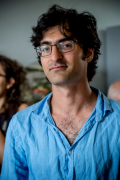Michael Goldman, Laboratoire Jacques-Louis Lions and Université Paris 7
On an old conjecture of Almgren

In this talk I will give an overview on the few results available on the conjecture of Almgren regarding the convexity of drops subject to the action of an external potential. In particular I will present recent progress in this direction obtained with G. De Philippis on their connectedness. Together with an older result of McCann, this answers positively the conjecture in dimension two. The proof is inspired by the two-point function technique introduced by B. Andrews and is reminiscent of the doubling of variables trick in the context of viscosity solutions.

talk_convex_MGoldman.pdf

Projecto FCT UIDB/04459/2020.

Projecto FCT UIDB/04459/2020.

## 05/05/2020, Tuesday, 16:00–17:00 Europe/Lisbon Room P1, Mathematics Building — OnlineFilippo Santambrogio, Université Claude Bernard - Lyon 1
Optimal transport methods for the regularity of 2D functions of least gradient

The least gradient problem (minimizing the BV norm with given boundary data), motivated by both image processing applications and connections with minimal surfaces, is known to be equivalent, in the plane, to the Beckmann minimal-flow problem (an alternative formulation of the $L^1$ Monge-Kantorovich optimal transport problem) with source and target measures located on the boundary of the domain. Hence, Sobolev regularity of functions of least gradient is equivalent in this setting to $L^p$ bounds on the solution of the Beckmann problem (i.e. on what is called the transport density) and can be attacked with techniques which are now standard in optimal transport. From the transport point of view, the novelty of the estimates that I will present, coming from a joint paper with S. Dweik, lies in the fact they are obtained for transport between measures which are concentrated on the boundary. From the BV point of view, a new result is the $W^{1,p}$ regularity of the least gradient function whenever the boundary datum is $W^{1,p}$ as a $1D$ function: moreover, the optimal transport framework is strong enough to deal with arbitrary strictly convex norms instead of the Euclidean one with almost no effort.

Projecto FCT UIDB/04459/2020.

Projecto FCT UIDB/04459/2020.

## 12/05/2020, Tuesday, 16:30–17:30 Europe/Lisbon Room P1, Mathematics Building — OnlineDiogo Oliveira e Silva, University of Birmingham
Global maximizers for spherical restriction

We prove that constant functions are the unique real-valued maximizers for all $L^2-L^{2n}$ adjoint Fourier restriction inequalities on the unit sphere $\mathbb{S}^{d-1}\subset\mathbb{R}^d$, $d\in\{3,4,5,6,7\}$, where $n\geq 3$ is an integer. The proof uses tools from probability theory, Lie theory, functional analysis, and the theory of special functions. It also relies on general solutions of the underlying Euler-Lagrange equation being smooth, a fact of independent interest which we discuss. We further show that complex-valued maximizers coincide with nonnegative maximizers multiplied by the character $e^{i\xi\cdot\omega}$, for some $\xi$, thereby extending previous work of Christ & Shao (2012) to arbitrary dimensions $d\geq 2$ and general even exponents. This talk is based on results obtained with René Quilodrán.

Global maximizers.pdf

Projecto FCT UIDB/04459/2020.

Projecto FCT UIDB/04459/2020.

## 19/05/2020, Tuesday, 16:00–17:00 Europe/Lisbon Room P1, Mathematics Building — OnlineBernold Fiedler, Institute of Mathematics, Freie Universität Berlin
Sturm meanders: global attractors, Temperley-Lieb algebras, and black holes

Fusco and Rocha studied Neumann boundary value problems for scalar ODEs of second order via a shooting approach. They introduced the notion of what we now call Sturm permutations. These permutations relate, on the one hand, to a special class of meandering curves as introduced by Arnol’d in a singularity theory context. On the other hand, they became central in the study of global attractors of nonlinear parabolic partial differential equations of Sturm type.

We discuss relations of Sturm meanders with further areas: the multiplicative and trace structure in Temperley-Lieb algebras, discrete versions of Cartesian billiards, and the problem of constructing initial conditions for black hole dynamics which satisfy the Einstein constraints. We also risk a brief glimpse at the long and meandric history of meander patterns themselves.

We report on joint work with Pablo Castañeda, Juliette Hell, Carlos Rocha, and Brian Smith. See also http://dynamics.mi.fu-berlin.de/

For further material we recommend the beautifully illustrated book “Meanders” by Anna Karnauhova, de Gruyter 2017.

Projecto FCT UIDB/04459/2020.

Projecto FCT UIDB/04459/2020.

## 26/05/2020, Tuesday, 16:00–17:00 Europe/Lisbon OnlineLuis Vega, Basque Center for Applied Mathematics
The Vortex Filament Equation, the Talbot effect, and non-circular jets.

We will propose the vortex filament equation as a possible toy model for turbulence, in particular because of its striking similarity to the dynamics of non-circular jets. This similarity implies the existence of some type of Talbot effect due to the interaction of non-linear waves that propagate along the filament. Another consequence of this interaction is the existence of a new class of multi-fractal sets that can be seen as a generalization of the graph of Riemann’s non-differentiable function. Theoretical and numerical arguments about the transfer of energy will be also given. This a joint work with V. Banica and F. de la Hoz.

Projecto FCT UIDB/04459/2020.

Projecto FCT UIDB/04459/2020.

## 02/06/2020, Tuesday, 16:00–17:00 Europe/Lisbon Room P1, Mathematics Building — OnlineMaria Colombo, École Polytechnique Fédérale de Lausanne
Nonunique characteristic curves of Sobolev vector fields

Given a vector field in $\mathbb{R}^d$, the classical Cauchy-Lipschitz theorem shows existence and uniqueness of its flow provided the vector field is sufficiently smooth; this, in turn, translates in existence and uniqueness results for the transport equation. In 1989, Di Perna and Lions proved that Sobolev regularity for vector fields, with bounded divergence and a growth assumption, is sufficient to establish existence, uniqueness and stability of a generalized notion of flow, consisting of a suitable selection among the trajectories of the associated ODE. A long-standing open question is whether the uniqueness of the regular Lagrangian flow is a corollary of the uniqueness of the trajectory of the ODE for a.e. initial datum. In this talk we give an overview of the topic and we provide a negative answer to this question. To show this result we exploit the connection with the transport equation, based on Ambrosio’s superposition principle, and a new ill-posedness result for positive solutions of the continuity equation.

ColomboTransport.pdf

The last webinars are already available at our youtube channel.

Projecto FCT UIDB/04459/2020.

Projecto FCT UIDB/04459/2020.

## 09/06/2020, Tuesday, 16:00–17:00 Europe/Lisbon Room P1, Mathematics Building — OnlineLucio Boccardo, Università di Roma La Sapienza
Recent developments on Dirichlet problems with singular convection/drift terms

In the talk we discuss two Dirichlet problems ("formally" in duality)\begin{align}\label{(CP)}\tag{CP}u\in W_0^{1,q}(\Omega): \; &\operatorname{div}(M(x)\nabla {u})+a(x)\,{u}=-\operatorname{div}({u}\,E(x)) +f(x);
\\ \label{(DP)}\tag{DP}\psi\in W_0^{1,q}(\Omega): & - \operatorname{div}(M(x)\nabla \psi)+a(x)\,\psi= E(x)\cdot\nabla \psi +g(x)\end{align}where $\Omega$ is a bounded open set in $\mathbb{R}^N$, $M(x)$ ia bounded elliptic matrix, $f$, $g$ are functions belonging to $L^m(\Omega)$, $m\geq1$, $E\in(L^N(\Omega))^N$, $0\lt \alpha_0\leq a(x)\in L^1(\Omega)$.

In the first part we briefly recall some recent results:

• existence and summability properties of weak solutions ($q=2$), if $m\geq\frac{2N}{ N+1}$;
• Calderon-Zygmund theory ($q=m^*$, infinite energy solutions), if $1\lt m \lt \frac{2N}{ N+1}$;
• uniqueness;
• the case $|E|\leq\frac{A}{|x|}$, where $E\not\in(L^N(\Omega))^N$;
• the case $E\in(L^2(\Omega))^N$.

Then we show:

• a new (simpler) existence proof, thanks to the presence of the zero order term, for \eqref{(CP)};
• a straight duality proof for \eqref{(DP)};
• continuous dependence of the solutions with respect to the weak convergence of the coefficients;
• regularizing effect of dominated coefficients ($|f|\leq Q\,a(x)$ or $|g|\leq Q\,a(x)$, $Q\gt 0$);
• "weak" maximum principle: if $f(x)\geq0$ [$g(x)\geq0$] and, of course, not =0 a.e., then $u(x)\geq0$ [$\psi(x)\geq0$] and the set where $u$ [$\psi$] is zero has zero measure (at most).

Work in progress: obstacle problem; nonlinear principal part.
Open problem: "strong" maximum principle.

Projecto FCT UIDB/04459/2020.

Projecto FCT UIDB/04459/2020.

## 16/06/2020, Tuesday, 16:00–17:00 Europe/Lisbon Room P1, Mathematics Building — Online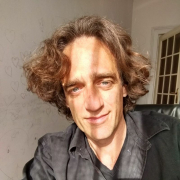Riccardo Adami, Politecnico di Torino
Ground states of the Nonlinear Schroedinger Equation on Graphs: an overview

Driven by physical and technological applications, during the last five years the study of nonlinear evolution on branched structures (graphs, networks) has undergone a fast development. We review on the main achievements and on the open problems. This is a joint project with several people, among which Simone Dovetta, Enrico Serra, Lorenzo Tentarelli, and Paolo Tilli.

The videos of the talks by Maria Colombo and Lucio Boccardo are already online on our youtube channel.

Projecto FCT UIDB/04459/2020.

Projecto FCT UIDB/04459/2020.

## 23/06/2020, Tuesday, 11:00–12:00 Europe/Lisbon OnlineTatsuya Miura, Tokyo Institute of Technology
On the isoperimetric inequality and surface diffusion flow for multiply winding curves

In this talk we discuss dynamical stability of multiply covered circles under the surface diffusion flow. To this end we first establish a general form of the isoperimetric inequality for immersed closed curves under rotational symmetry, which would be of independent interest. We then apply it to obtaining a certain class of rotationally symmetric initial curves from which solutions to the surface diffusion flow exist globally-in-time and converge to multiply covered circles. This talk is based on a joint work with Shinya Okabe at Tohoku University.

Slides_Miura.pdf

Please note that this seminar is at 11:00 Lisbon time, which is not the usual schedule.

The video of Riccardo Adami's talk is already available on youtube.

Projecto FCT UIDB/04459/2020.

Projecto FCT UIDB/04459/2020.

## 30/06/2020, Tuesday, 16:00–17:00 Europe/Lisbon Online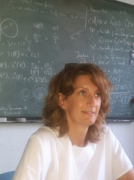Barbara Brandolini, Università Degli Studi di Napoli Federico II
Sharp lower bounds for Neumann eigenvalues

We will discuss lower bounds for the first non-trivial Neumann eigenvalue $\mu_1(\Omega)$ of the $p$-Laplace operator ($p \gt 1$) in a Lipschitz, bounded domain $\Omega$ in $\mathbb{R}^n$. In 1960 Payne and Weinberger proved that, when $\Omega$ is convex and $p = 2$, then \begin{equation}\label{eq:1}\mu_1(\Omega) \geq \frac{\pi^2}{d(\Omega)^2},\end{equation} where $d(\Omega)$ is the diameter of $\Omega$. The above estimate is asymptotically sharp, since $\mu_1(\Omega)d(\Omega)^2$ tends to $\pi^2$ for a parallelepiped all but one of whose dimensions shrink to $0$. On the other hand, it does not hold true in general for non-convex sets. In this talk we will focus on the non-convex setting. We will consider an arbitrary Lipschitz, bounded domain $\Omega$ in $\mathbb{R}^n$ and we will show a sharp lower bound for $\mu_1(\Omega)$ which, differently from \eqref{eq:1}, involves the best isoperimetric constant relative to $\Omega$ and is sharp, at least when $p = n = 2$, as the isoperimetric constant relative to $\Omega$ goes to $0$. Moreover, in a suitable class of convex planar domains, our estimate will turn out to be better than \eqref{eq:1}.

Furthermore, we will see that, when $p = n = 2$ and $\Omega$ consists of the points on one side of a smooth curve $\gamma$, within a suitable distance $\delta$ from it, then $\mu_1(\Omega)$ can be sharply estimated from below in terms of the length of $\gamma$, the $L^\infty$ norm of its curvature and $\delta$.

Further Information:

The slides of the last talk, by Tatsuya Miura, can be found here.

Other upcoming talks: Elvira Zappale (July 9 , 4pm) and Pavel Exner (July 14, 4pm).

Projecto FCT UIDB/04459/2020.

Projecto FCT UIDB/04459/2020.

## 09/07/2020, Thursday, 16:00–17:00 Europe/Lisbon Room P1, Mathematics Building — OnlineElvira Zappale, Università Degli Studi di Salerno
Optimal design problems

I will present several integral representation results for certain functionals arising in the context of optimal design and damage models, in presence of a perimeter penalization term. I will consider several frameworks, and I will also discuss the case with non-standard growth conditions.

Projecto FCT UIDB/04459/2020.

Projecto FCT UIDB/04459/2020.

## 14/07/2020, Tuesday, 16:00–17:00 Europe/Lisbon Room P1, Mathematics Building — Online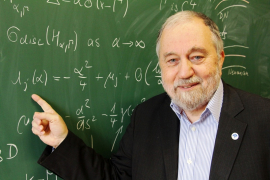Pavel Exner, Doppler Institute for Mathematical Physics and Applied Mathematics, Prague
Vertex coupling and spectra of periodic quantum graphs

The talk focuses on the influence of the vertex coupling on spectral properties of periodic quantum graphs. Specifically, two questions will be addressed. The first concerns the number of open spectral gaps; it is shown that graphs with a nontrivial $\delta$ coupling can have finite but nonzero number of them. Secondly, motivated by recent attempts to model the anomalous Hall effect, we investigate a class of vertex couplings that violate the time reversal invariance. For the simplest coupling of this type we show that its high-energy properties depend on the parity of the lattice vertices, and discuss various consequences of this property.

PExner_lisbon20w.pdf

Projecto FCT UIDB/04459/2020.

Projecto FCT UIDB/04459/2020.

## 04/09/2020, Friday, 11:00–12:00 Europe/Lisbon Room P1, Mathematics Building — Online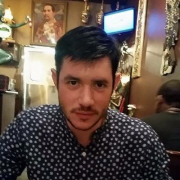Marco Morandotti, Politecnico di Torino
Spatially inhomogeneous evolutionary games

We study an interaction model of a large population of players based on an evolutionary game, which describes the dynamical process of how the distribution of strategies changes in time according to their individual success.

We assume that the population of players is distributed over a state space and that they are each endowed with probability distributions of pure strategies, which they draw at random to evolve their states. Simultaneously, the mixed strategies evolve according to a replicator dynamics, modeling the success of pure strategies according to a payoff functional.

We establish existence, uniqueness, and stability of Lagrangian and Eulerian solutions of this dynamical game by using methods of ODE and optimal transport on Banach spaces.

We apply the general theoretical framework to perform the mean-field analysis of a multi-population agent-based model, where a particle dynamics derived by a nonlocal velocity and a Markov-type jump process for the labels are coupled.

An alternate Lagrangian approximation scheme at discrete times is also proposed.

This research is from joint works with S. Almi, L. Ambrosio, M. Fornasier, G. Savaré, and F. Solombrino.

Projecto FCT UIDB/04459/2020.

Projecto FCT UIDB/04459/2020.

## 22/10/2020, Thursday, 14:30–15:30 Europe/Lisbon Room P1, Mathematics Building — OnlineJean-Baptiste Casteras, CMAFcIO, Universidade de Lisboa
Standing wave and travelling wave solutions for a fourth order Schrödinger equation

In this talk, we will be interested in standing wave solutions to a fourth order nonlinear Schrödinger equation having second and fourth order dispersion terms. This kind of equation naturally appears in nonlinear optics. In a first time, we will establish the existence of ground-state and renormalized solutions. We will then be interested in their qualitative properties, in particular their stability.

Joint works with Denis Bonheure, Ederson Moreira Dos Santos, Tianxiang Gou, Louis Jeanjean and Robson Nascimento.

Projecto FCT UIDB/04459/2020.

Projecto FCT UIDB/04459/2020.

## 29/10/2020, Thursday, 13:30–14:30 Europe/Lisbon Room P1, Mathematics Building — OnlineLorenzo Mazzieri and Piotr Chruściel, Università di Trento / University of Vienna
Static vacuum black holes and Lambda

We will review the status of the uniqueness theory of static vacuum black holes, with or without a cosmological constant $\Lambda$, and we will outline the proof of a uniqueness theorem with $\Lambda \gt 0$, proved jointly in collaboration with Stefano Borghini.

Projecto FCT UIDB/04459/2020.

Projecto FCT UIDB/04459/2020.

## 05/11/2020, Thursday, 14:30–15:30 Europe/Lisbon Room P1, Mathematics Building — OnlineWladimir Neves, Universidade Federal do Rio de Janeiro
Homogenization of Schrödinger equations. Extended Effective Mass Theorems for non-crystalline matter.

In this talk, we study the homogenization of the Schrödinger equation beyond the periodic setting. More precisely, rigorous derivation of the effective mass theorems in solid state physics for non-crystalline materials are obtained.

This is a joint work with Vernny Ccajma and Jean Silva.

Projecto FCT UIDB/04459/2020.

## 12/11/2020, Thursday, 14:30–15:30 Europe/Lisbon Room P1, Mathematics Building — OnlineRainer Mandel, Karlsruhe Institute of Technology
New dual variational methods for Nonlinear Helmholtz Equations and polychromatic solutions of Nonlinear wave equations

In the first part of my talk, I present the classical dual variational method in the context of Nonlinear Helmholtz equations that describe monochromatic waves in nonlinear materials. Afterwards, I discuss two recent generalizations of the method. The first deals with an extension to Nonlinear Helmholtz equations with sign-changing nonlinearities. For these problems we construct solutions that have infinite Morse-Index in the dual variational formulation. The second generalization concerns dual variational methods for the construction of breathers, i.e., polychromatic, spatially localized and time-periodic solutions of nonlinear wave equations.

The results were obtained in collaboration with D. Scheider and T. Yesil.

Projecto FCT UIDB/04459/2020.

## 16/11/2020, Monday, 14:30–15:30 Europe/Lisbon Room P1, Mathematics Building — OnlineHynek Kovařík, Università degli studi di Brescia
Absence of positive eigenvalues of magnetic Schroedinger operators

We study sufficient conditions for the absence of positive eigenvalues of magnetic Schroedinger operators in $\mathbb{R}^n$. In our main result we prove the absence of eigenvalues above certain threshold energy which depends explicitly on the magnetic and electric field. A comparison with the examples of Miller-Simon shows that our result is sharp as far as the decay of the magnetic field is concerned.

The talk is based on a joint work with Silvana Avramska-Lukarska and Dirk Hundertmark.

lisbon-2020-kovarik.pdf

**Please note that this seminar will take place Monday, not the usual day of the Lisbon WADE.**

Partially supported by project FCT UIDB/04459/2020.

## 26/11/2020, Thursday, 13:30–14:30 Europe/Lisbon Online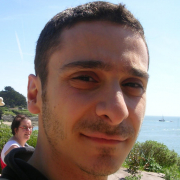Jean-Francois Babadjian, Université Paris Saclay
Concentration versus oscillation effects in brittle damage

This talk is concerned with the asymptotic analysis of a variational model of brittle damage, when the damaged zone concentrates into a set of zero Lebesgue measure, and, at the same time, the stiffness of the damaged material becomes arbitrarily small. In a particular non-trivial regime, concentration leads to a limit energy with linear growth as typically encountered in perfect plasticity. While the singular part of the limit energy can be easily described, the identification of the bulk part of the limit energy requires a subtler analysis of the interplay between concentration and oscillation properties of the displacements.

This is a joint work with F. Iurlano and F. Rindler.

## 26/11/2020, Thursday, 14:30–15:30 Europe/Lisbon Room P1, Mathematics Building — OnlineMarco Caroccia, Università degli studi di Roma Tor Vergata
Contact surface of Cheeger sets

Geometrical properties of Cheeger sets have been deeply studied by many authors since their introduction, as a way of bounding from below the first Dirichlet (p)-Laplacian eigenvualue. They represents the first eigenfunction of the Dirichlet (1)-Laplacian of a domain. In this talk we will introduce a recent property, studied in collaboration with Simone Ciani (Università degli studi di Firenze), concerning their contact surface with the ambient space. In particular we will show that the contact surface cannot be too small, with a lower bound on the dimension strictly related to the regularity of the ambient space. The talk will focus on the introduction of the problem. It will start with a brief explanation of its connection with the Dirichlet (p)-Laplacian eigenvalue problem. Then a brief sketch of the proof is given. Functional to the whole argument is the notion of removable singularity, as a tool for extending solutions of pdes under some regularity constraint.

## 03/12/2020, Thursday, 14:30–15:30 Europe/Lisbon Online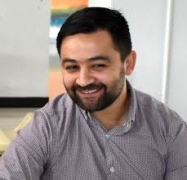Youcef Mammeri, Université de Picardie Jules Verne
A SIR-type model with diffusion to describe the spatial spread of Covid-19

We all have to deal with the coronavirus epidemic. Many strategies have been put in place to try to contain the disease, with varying success.

I will present an SIR-type mathematical model to predict the state of the epidemic. The effect of distancing, isolation of exposed individuals and treatment of symptoms will be compared.

I will begin with a simple explanation of SIR models, then discuss a PDE model and its resolution.

Projecto FCT UIDB/04459/2020.

## 10/12/2020, Thursday, 14:30–15:30 Europe/Lisbon Room P1, Mathematics Building — OnlineQiao Huang, GFM, Universidade de Lisboa
Stochastic Cucker-Smale model: collision-avoidance and flocking

In this talk, we consider the Cucker-Smale flocking model involving both singularity and noise. We first show the local strong well-posedness for the system, in which the communication weight is locally Lipschitz beyond the origin. Then, for the special case that the communication weight has a strong singularity at the origin, we establish the global well-posedness by showing the finite time collision-avoidance. Finally, we study the large time behavior of the system when the communication weight is of zero lower bound. The conditional flocking emerges for the case of constant noise intensity, while the unconditional flocking emerges for various time-varying intensities and long-range communications.

## 17/12/2020, Thursday, 14:30–15:30 Europe/Lisbon Room P1, Mathematics Building — Online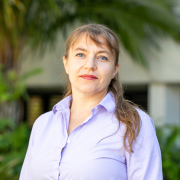Svetlana Roudenko, Florida International University
Zakharov-Kuznetsov equation: toward soliton resolution

We consider Zakharov-Kuznetsov (ZK) equation, which is a higher-dimensional version of the Korteweg-de Vries (KdV) equation, and investigate the dynamics of solutions, especially questions about the soliton stability. We first discuss the situation in two dimensions, in particular, the instability of solitons in the 2d cubic (critical) ZK equation, which leads to blow-up. Then we consider the 3d quadratic ZK equation, originally introduced by Zakharov and Kuznetsov in early 1970's, and discuss the asymptotic stability of solitons. We will also show numerical findings on the formation of solitons and radiation in this equation. This talk will be based on joint works with L.G. Farah, J. Holmer, C. Klein, N. Stoilov, K. Yang.

Projecto FCT UIDB/04459/2020.